## Friday, January 21, 2011

### Building Junction Trees

Here's a 367 vertex Apollonian Network and its Junction Tree (aka Tree Decomposition)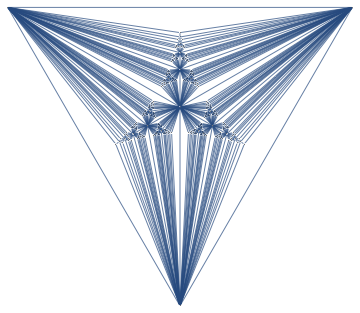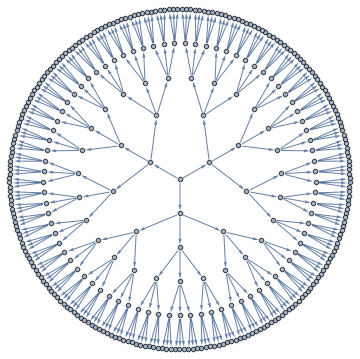A Junction Tree provides an efficient data structure to do exact probabilistic inference. In the context of traditional graph algorithms, it is known as the "Tree Decomposition". The amount of structure apparent from the junction tree shows that problems structured as Apollonian Networks have very low computational complexity.

The same junction tree structure can also be used to efficiently compute chromatic polynomials, find cliques, count self-avoiding walks, and the reason for this universality is that it essentially captures separation structure of the graph -- it is a representation of a complete set of vertex separators. In the Junction Tree above you can see 121 non-leaf nodes that separate the graph into 3 parts. Each node corresponds to a set of 4 vertices. There are also 363 edges, each corresponds to a set of 3 vertices that separates the graph into 2 parts. The fact that the graph can be recursively partitioned using small separators implies an efficient recursion scheme for problems on this graph.

I put together a Mathematica package to build Junction Trees like above using MinFill, MinCut and a few other heuristics. Some experimenting showed MinFill with Vertex Eccentricity for choosing between vertices with equal fill to work the best for general graphs, whereas MinCut worked the best for planar graphs.

## Friday, January 14, 2011

### P vs. NP page

Here's a page linking 65 attempts of resolving P vs NP problem. A couple of papers were published in peer-reviewed journals or conferences, while most are "arxiv" published. Some statistics:

• 35 prove P=NP
• 28 prove P!=NP
• 2 prove it can go either way (undecidable)

## Saturday, January 08, 2011

### towards Problem Compilers

First programmers wrote in machine code and assemblers simplified this task significantly by letting them give algorithms at a higher level. I still find stacks of punch cards like below in my St.Petersburg home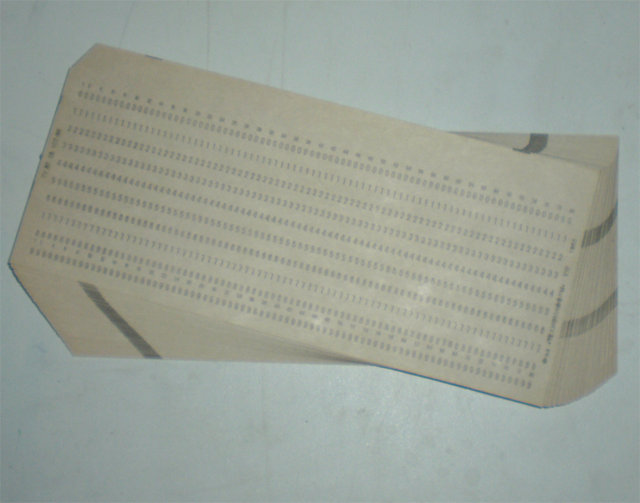Wouldn't it be great if we could extend this idea further and have the computer compile the problem into machine code?

Actually, we already have such tools restricted to various versions of "problem language". For instance if you express your problem in terms of integer optimization, you can use integer programming tools.

Another language is the language of "graphical models". In the most general formulation, you specify meaning of operations + and *, that obey distributive law, set of x variables, set of functions f, and ask computer to find the following

$$\bigoplus_{x_1,x_2,\ldots} \bigotimes_f f(x)$$

This formulation has a natural "interactions graph" associated with it, and a computer can find an algorithm to solve this problem using tree decomposition if interactions between f functions are not too complex

However, this is still suboptimal because the algorithm designer needs to pick variables x and functions f, and this choice is fairly important. For instance, for minimum weight Steiner Tree, "natural" variables of the problem don't give a good factorization, and you need a smart designer to figure out a good reparameterization of the problem, like here.

As it stands right now, if a designer gives a good parameterization of the problem, a computer can solve it. Can we train a computer to find a parameterization?

I don't know the answer, but one potential approach to finding parameterizations is described in A new look at Generalized Distributive Law. They formulate the problem of optimal decomposition as that of search at the level of individual instantiations of x, so theoretically, this approach can discover optimal parameterization of the problem.

## Sunday, January 02, 2011

### Interactive Tree Decomposition

Here's a tool (in Mathematica) to help visualize the process of constructing a Junction Tree. wrong link fixed

Clicking on vertices corresponds to vertex elimination and each click creates a new bag. After all vertices are eliminated, junction tree is created by removing redundant bags and taking the maximum spanning tree with size of the separators defining the weight of each edge as the junction tree.

If you try it on small examples, you can get some intuition of how outperform the greedy approach -- basically you want to find small separators of the graph, and at the same time you want those separators to have a lot of vertices in common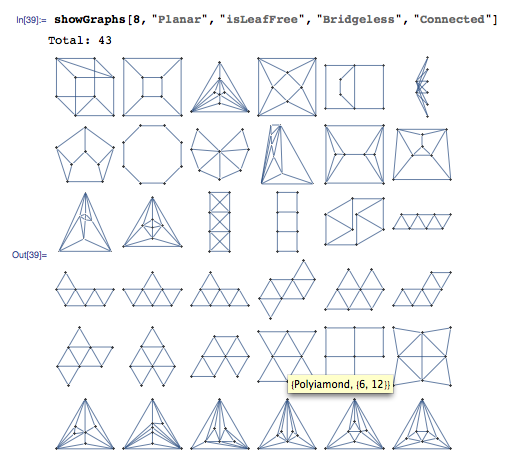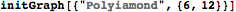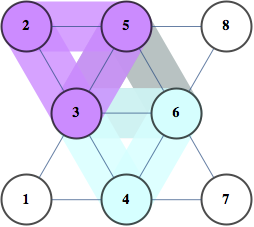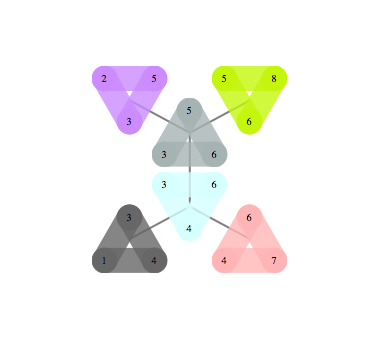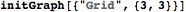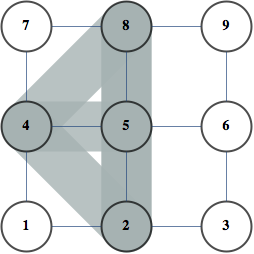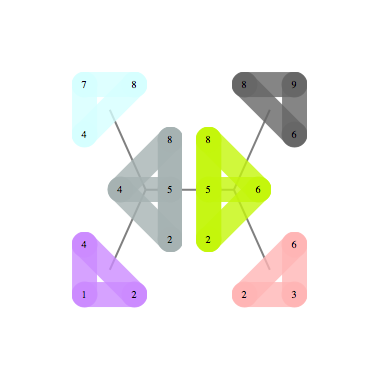## Saturday, January 01, 2011

### Happy New Year

Here are some links to start the new year on a light note

• Hinged tesselation

• Statistics-related Cartoons on stats.SE• Memorable math paper titles, like Coxeter's "My Graph" (about Coxeter graph).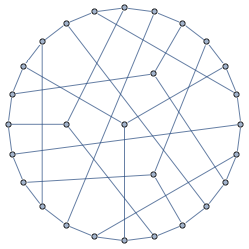• Memorable Computer Science paper titles. It includes a series of papers "Functional Programming with Bananas, Lenses, Envelopes and Barbed Wire", and "Seee More through Lenses than Bananas.". Apparently these are functional programming terms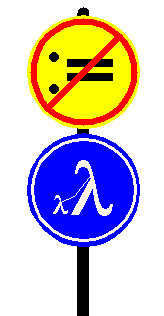• Annals of Improbable Research